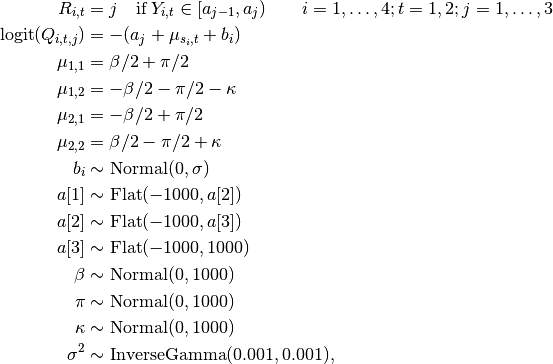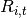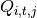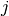# Inhalers: Ordered Categorical Data¶

An example from OpenBUGS  and Ezzet and Whitehead  concerning a two-treatment, two-period crossover trial comparing salbutamol inhalation devices in 286 asthma patients.

## Model¶

Treatment responses are modelled aswhereis a 4-point ordinal rating of the device used by patient, andis the cumulative probability of the rating in treatment periodbeing worse than category.

## Analysis Program¶

using Mamba

## Data
inhalers = Dict{Symbol, Any}(
:pattern =>
[1 1 1 1 2 2 2 2 3 3 3 3 4 4 4 4
1 2 3 4 1 2 3 4 1 2 3 4 1 2 3 4]',
:Ncum =>
[ 59 157 173 175 186 253 270 271 271 278 280 281 282 285 285 286
122 170 173 175 226 268 270 271 278 280 281 281 284 285 286 286]',
:treat =>
[ 1 -1
-1  1],
:period =>
[1 -1
1 -1],
:carry =>
[0 -1
0  1],
:N => 286,
:T => 2,
:G => 2,
:Npattern => 16,
:Ncut => 3
)

inhalers[:group] = Array{Int}(inhalers[:N])
inhalers[:response] = Array{Int}(inhalers[:N], inhalers[:T])

i = 1
for k in 1:inhalers[:Npattern], g in 1:inhalers[:G]
while i <= inhalers[:Ncum][k, g]
inhalers[:group][i] = g
for t in 1:inhalers[:T]
inhalers[:response][i, t] = inhalers[:pattern][k, t]
end
i += 1
end
end

## Model Specification
model = Model(

response = Stochastic(2,
(a1, a2, a3, mu, group, b, N, T) ->
begin
a = Float64[a1, a2, a3]
UnivariateDistribution[
begin
eta = mu[group[i], t] + b[i]
p = ones(4)
for j in 1:3
Q = invlogit(-(a[j] + eta))
p[j] -= Q
p[j + 1] = Q
end
Categorical(p)
end
for i in 1:N, t in 1:T
]
end,
false
),

mu = Logical(2,
(beta, treat, pi, period, kappa, carry, G, T) ->
[ beta * treat[g, t] / 2 + pi * period[g, t] / 2 + kappa * carry[g, t]
for g in 1:G, t in 1:T ],
false
),

b = Stochastic(1,
s2 -> Normal(0, sqrt(s2)),
false
),

a1 = Stochastic(
a2 -> Truncated(Flat(), -1000, a2)
),

a2 = Stochastic(
a3 -> Truncated(Flat(), -1000, a3)
),

a3 = Stochastic(
() -> Truncated(Flat(), -1000, 1000)
),

beta = Stochastic(
() -> Normal(0, 1000)
),

pi = Stochastic(
() -> Normal(0, 1000)
),

kappa = Stochastic(
() -> Normal(0, 1000)
),

s2 = Stochastic(
() -> InverseGamma(0.001, 0.001)
)

)

## Initial Values
inits = [
Dict(:response => inhalers[:response], :beta => 0, :pi => 0, :kappa => 0,
:a1 => 2, :a2 => 3, :a3 => 4, :s2 => 1, :b => zeros(inhalers[:N])),
Dict(:response => inhalers[:response], :beta => 1, :pi => 1, :kappa => 0,
:a1 => 3, :a2 => 4, :a3 => 5, :s2 => 10, :b => zeros(inhalers[:N]))
]

## Sampling Scheme
scheme = [AMWG(:b, 0.1),
Slice([:a1, :a2, :a3], 2.0),
Slice([:beta, :pi, :kappa, :s2], 1.0, Univariate)]
setsamplers!(model, scheme)

## MCMC Simulations
sim = mcmc(model, inhalers, inits, 5000, burnin=1000, thin=2, chains=2)
describe(sim)